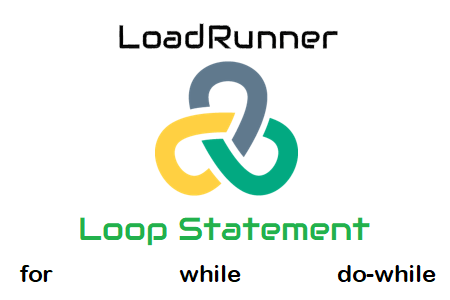The reason behind to add a loop statement in the LoadRunner script is to implement the repetitive code logic. In LoadRunner script, if you want to send a particular request for X number of times then there are two ways. One way is to write the request X number of times so that LoadRunner can send it to the server. Another way is to insert a loop in the script and add the request under that loop. The loop will run X times and send the request in a repetitive manner.

To understand how does a loop work, you need to know the logic. A loop statement comprises of 3 parts; initialization, condition and increment/decrement. The initialization part initializes a variable and assigns a value, the condition part compares the value of the variable with a given condition and decides whether to continue or break the loop. The last part increases or decreases the value of the variable. Let’s try to understand with an example:

#### Example:

Considering a simple ‘for’ loop statement has a loop variable i of type integer. You want to execute the loop for 3 times and then exit. The ‘for’ loop statement will be:

``````int i;
for (i=1;i<=3;i++) // Loop Body
{
web_url(...) //Request
}``````

i=1 => Assign the initial value of i as 1.
i<=3 => Evaluate the value of i before starting the loop. The value of i should be less than or equal to 3.
i++ => Increase the value of i by 1 after the completion of each loop

#### How does a loop work?

Loop 1: The initial value of i is 1 which satisfies the condition i<=3. Hence the loop body executes and the request is sent to the server. The value of i will be increased by 1 (i++) and became 2 (i=2).

Loop 2: Since the value of i becomes 2 (i=2) in the previous loop, so no initialization will occur. Now, evaluate the condition i<=3 which is true. Hence again request will be sent to the server for the second time. Due to increment logic (i++), the value of i becomes 3 (i=3).

Loop 3: The value of i becomes 3 (i=3) in the previous loop. Evaluate the condition i<=3 which is true. Again the request will be sent to the server for the third time. The value of i becomes 4 (i=4).

Loop 4: Now, the value of i becomes 4 (i=4) in the previous loop. Evaluate the condition i<=3 which does not satisfy. Hence the control will exit the loop without sending the request to the server.

The above loop has sent the request 3 times to the server and fulfils the purpose.

Basically, there are 3 types of loop statements in the LoadRunner which are: